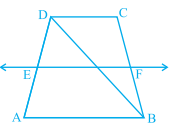"
">

# $\mathrm{ABCD}$ is a trapezium in which $\mathrm{AB} \| \mathrm{DC}, \mathrm{BD}$ is a diagonal and $\mathrm{E}$ is the mid-point of $\mathrm{AD}$. A line is drawn through E parallel to $\mathrm{AB}$ intersecting $\mathrm{BC}$ at $\mathrm{F}$ (see below figure). Show that $\mathrm{F}$ is the mid-point of $\mathrm{BC}$."

Given:

$\mathrm{ABCD}$ is a trapezium in which $\mathrm{AB} \| \mathrm{DC}, \mathrm{BD}$ is a diagonal and $\mathrm{E}$ is the mid-point of $\mathrm{AD}$.

A line is drawn through E parallel to $\mathrm{AB}$ intersecting $\mathrm{BC}$ at $\mathrm{F}$

To do:
We have to show that $\mathrm{F}$ is the mid-point of $\mathrm{BC}$.

Solution:

Let $P$ be the point of intersection of $BD$ and $EF$.

In $\triangle ABD$,

$EP \| AB$

$E$ is the mid-point of $AD$.

We know that,

A line drawn through the mid-point of one side of a triangle parallel to another side bisects the third side.

Therefore,

$P$ is the mid-point of $BD$.

In $\triangle BCD$,

$PF \| CD$

$P$ is the mid-point of $BD$.

By converse of mid-point theorem,

$F$ is the mid-point of $CB$.

Hence proved.

Updated on: 10-Oct-2022

24 Views